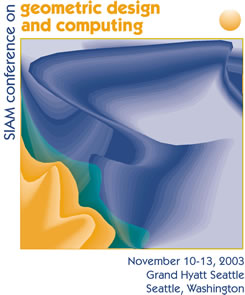### SIAM Short Course on Meshfree Modeling with Distances and Splines

November 9, 2003

Organizers: Ulrich Reif, Technische Universität Darmstadt, Germany

### Rationale

Many problems in geometric design and engineering analysis are dominated by spatial discretization of the geometric domain into a conforming grid of simple elements (mesh). This tutorial will demonstrate that many such problems may be formulated and solved without explicit meshing, using approximate distance fields and splines.

### Lecturers

Ulrich Reif (http://www.mathematik.tu-darmstadt.de/~reif/) is a Professor at the Department of Mathematics at Darmstadt University of Technoloy since 2000. He studied mathematics and electrical engineering at Stuttgart University, where received his PhD in 1993 and his Habilitation in 1999. His fields of interest include Geometric Modeling, Subdivision algorithms, and spline approximation. Recent work addresses finite element simulations with weighted extended B-splines.

Vadim Shapiro (http://www.engr.wisc.edu/me/faculty/shapiro_vadim.html) is a Professor of Mechanical Engineering and Computer Sciences at the University of Wisconsin-Madison where he has been on faculty since 1994. Prior to that he was a member of research staff at the General Motors R&D Center working in the areas of geometric modeling and design automation. He received BA degrees in mathematics and in computer science from New York University, MS in computer science from UCLA, and MS and PhD in mechanical engineering from Cornell University. In addition to solid modeling, his research interests include unification of physical and geometric representations and computational design.

Igor Tsukanov (http://sal-cnc.me.wisc.edu/~igor/igor.html) is a research scientist at the Department of Mechanical Engineering of University of Wisconsin-Madison working on automation of the R-function meshfree method. He received his Ph.D. degree in 1997 from the Ukrainian National Academy of Sciences. From 1992 till 1998 he worked in the research group headed by Prof. Rvachev at Institute for Problems in Machinery of Ukrainian National Academy of Sciences. In 1998 he joined Spatial Automation Laboratory at UW-Madison. Research interests include meshfree methods of engineering analysis, numerical methods for PDE's, automation.

### Course Description

The approach to modeling relies on the observation that any field problem may be modeled as a function of weighted distances to a given collection of geometric features. In addition to various geometric applications, field-modeling applications include (heterogeneous) material modeling and meshfree solution of engineering analysis problems. The tutorial will consists of three parts:

1. Fields as functions of approximate distances. Every field function satisfying some given boundary conditions on a collection of geometric features may be put into a canonical form in terms of powers of distances to the feature. Once a distance field is known, the canonical form allows systematic construction, comparison, and approximation of fields satisfying boundary conditions without relying on meshing. Many different approximations of distance fields are known, but we will explore in details smooth approximations of distance fields using theory of R-functions originally developed by V. L. Rvachev.

2. Formulating and solving boundary value problems. Once the boundary conditions are satisfied in terms of distance fields, a solution to boundary value problem maybe constructed by a linear combination of appropriately weighted basis functions. In particular, weighted extended B-splines (web-splines) allow constructing efficient and numerically stable approximations on arbitrary domains.

3. Computational tools and applications. Effective applications of the above techniques require accurate and efficient computational procedures for sampling, differentiation, integration, and visualization of fields over solids and domains. We will discuss the required utilities, feasible solutions, and demonstrate a generic system for field modeling using approximate distance fields. Example applications include modeling of solids with heterogeneous materials and meshfree solution of various engineering analysis problems including heat transfer, vibration, stress analysis, and thermo-elasticity.

### Course Objectives

Attendees will be able to model and solve a variety of field problems without depending on meshing.

### Course Level

Introductory: 20% Intermediate: 60% Advanced: 20%